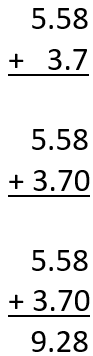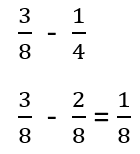# Math Worksheets Land

Math Worksheets For All Ages

# Math Worksheets Land

Math Worksheets For All Ages

Home > Math Topics > Decimals >

# Adding and Subtracting Decimals and Fractions Worksheets

By this time students have become quite comfortable process basic operations with whole numbers. What happens when we find values in different formats? The fundamentals of processing basic operations are still the same, there are just subtle differences that we must account for. We use decimal values to process calculations with money all the time. In the case of currency, the whole number values represent dollars, and the decimal portion represents cents. When we are making a recipe in our kitchens we will often need to add or subtract fractions to determine the amount of ingredients that we will need or when we do not have the properly measuring utensils. These lessons and worksheets have students learn how to be comfortable with adding or subtracting decimals and/or fractions.

### Aligned Standard: 5.NF.A.1 and 6.NS.B.3

• Answer Keys - These are for all the unlocked materials above.

### Homework Sheets

The first version has slightly easier problems to help students build confidence.

• Homework 1 - Find each sum or difference. Fraction answers must be proper and in simplest terms.
• Homework 2 - Decimals: Align the decimal points then add or subtract.

### Practice Worksheets

Problem number three can be written as an improper fraction or mixed number.

• Practice 2 - Fractions: Get common denominators, add or subtract, then simplify.

### Math Skill Quizzes

The difficulty of the quizzes is about the same. We really don't see problems that are aimed for this level that are at a higher level.

• Quiz 1 - Watch out for the negative values here. Evaluate those first.
• Quiz 2 - No hints here, get after it.

### Tips for Adding and Subtracting Decimals and FractionsApplying arithmetic operations, such as addition, subtraction, multiplication, and division, on whole numbers is easy and not that of a challenge. The students begin to face challenges when they have to apply the basic operations on values that are found in decimal or fractional. We both formats it is all about making sure that you keep everything aligned properly and form a sense of balance.

Adding Decimal Values - There are certain rules that students have to follow when adding decimal numbers. To become a pro at addition of decimal numbers, memorizing these rules prove very helpful.

1. Align - Position one addend above the other in a vertical format. Align their decimal points.

2. Proper Number of Zeroes - Always write the digits at the right place value. If two numbers do not have equal decimal digits, place zero where the digit ends.

3. Add Them Up - Add just like you add whole numbers. Start at the lowest place value and work your way up. The decimal point of all numbers must lie exactly below the first one.

Subtracting Decimal Values - This follows the same tips as we just explored in addition. We are just using the opposite operation.

Adding and Subtracting Fractions - Just like decimals, the process for finding the sum or difference of fractions follows the same steps, just opposite operations. Since we found a sum between decimals, lets find a difference between fractions using these tips:1. Like Denominators - Make sure that the denominators (bottom number) are the same on both the minuend and subtrahend. If they are not the same, find a common denominator and convert both values to an equivalent fraction with that new denominator.

2. Subtract Numerators - Subtract the top numbers, now that the denominators are the same the denominator continue to stay the same.

3. Reduce the Fraction - If needed simplify the fraction to its lowest form.

Unlock all the answers, worksheets, homework, tests and more!
Save Tons of Time! Make My Life Easier Now

## Thanks and Don't Forget To Tell Your Friends!

I would appreciate everyone letting me know if you find any errors. I'm getting a little older these days and my eyes are going. Please contact me, to let me know. I'll fix it ASAP.